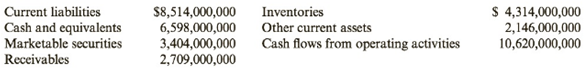Chapter 8, Problem 72E### Cornerstones of Financial Accounti...

4th Edition
Jay Rich + 1 other
ISBN: 9781337690881

#### Solutions

Chapter
Section### Cornerstones of Financial Accounti...

4th Edition
Jay Rich + 1 other
ISBN: 9781337690881
Textbook Problem
9 views

# Ratio AnalysisIntel Corporation provided the following information on its balance sheet and statement of cash flows:Required:1. Calculate the (a) current ratio, (b) quick ratio, (c) cash ratio, and (d) operating cash flow ratio. ( Note: Round answers to two decimal places.)2. CONCEPTUAL CONNECTION Interpret these results.3. CONCEPTUAL CONNECTION Assume that Intel, as a requirement of one of its loans, must maintain a current ratio of at least 2.30. Given the large amount of cash, how could Intel accomplish this on December 31 (be specific as to dollar amounts)?

To determine

(a)

Introduction:

Every investor or lender is concerned with the liquidity ratios of the company. It helps them analyze the company’s ability to meet short term obligations.

To calculate:

Liquidity Ratios

(a)Current ratio
(b)Quick ratio
(c)Cash ratio
(d)Operating cash flow ratio.

Explanation

(a) Current Ratio = Current AssetsCurrent Liabilities

Current Assets = Cash & Cash equivalents + Marketable Securities + Receivables + Inventories + Other Current Assets

Current Assets = $6,598,000,000 +$3,404,000,000 + $2,709,000,000 +$4,314,000,000 + $2,416,000,000 Current Assets =$19,441,000,000

Current Liabilities = 8,514,000,000

Current Ratio = $19,441,000,000$8,514,000,000

Current Ratio = 2.28

(b) Quick Ratio = Quick AssetsCurrent Liabilities

Quick Assets = Cash & Cash equivalents + Marketable Securities + Receivables

Quick Assets = $6,598,000,000 +$3,404,000,000 + $2,709,000,000 Quick Assets =$12,711,000,000

Current Liabilities = 8,514,000,000

Quick Ratio = $12,711,000,000$8,514,000,000

Quick Ratio = 1

To determine

(b)

Introduction:

Every investor or lender is concerned with the liquidity ratios of the company. It helps them analyze the company’s ability to meet short term obligations.

To interpret:

Liquidity Ratios.

To determine

(c)

Introduction:

Every investor or lender is concerned with the liquidity ratios of the company. It helps them analyze the company’s ability to meet short term obligations.

To discuss:

Accomplishment of desired current ratio.

### Still sussing out bartleby?

Check out a sample textbook solution.

See a sample solution

#### The Solution to Your Study Problems

Bartleby provides explanations to thousands of textbook problems written by our experts, many with advanced degrees!

Get Started

#### What is logical independence?

Database Systems: Design, Implementation, & Management

#### What does CBN stand for?

Precision Machining Technology (MindTap Course List)

#### Compute the interest earned on the deposits made in Problem 20.1.

Engineering Fundamentals: An Introduction to Engineering (MindTap Course List)

#### What is an evaluation model? How would you create a weighted evaluation model?

Systems Analysis and Design (Shelly Cashman Series) (MindTap Course List)

#### Differentiate between storage and memory and describe how they interact.

Enhanced Discovering Computers 2017 (Shelly Cashman Series) (MindTap Course List)